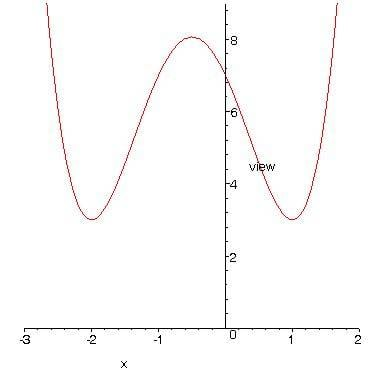# More than one tangent

## Homework Statement

Show that the tangent to the curve $$y=(x^2+x-2)^2+3$$ at the point where x =1 is also tangent to the curve at another point.

## Homework Equations

$$y=(x^2+x-2)^2+3$$

## The Attempt at a Solution

$$y'=2(x^2+x-2)(2x+1)$$
$$y'(1)=0$$
$$0=2(2x+1)(x+2)(x-1)$$

x= -1/2, -2, 1

Would this be correct?

Last edited:

## Homework Statement

Show that the tanget to the curve $$y=(x^2+x-2)^2+3$$ at the point where x =1 is also tangent to the curve at another point.

## Homework Equations

$$y=(x^2+x-2)^2+3$$

## The Attempt at a Solution

$$y'=2(x^2+x-2)(2x+1)$$
$$y'(1)=0$$
$$0=2(2x+1)(x+2)(x-1)$$

x= -1/2, -2, 1

Would this be correct?

Yes.

For $$f'(x) = 0$$, I also get x= -2 or x= -1/2 or x = 1.

Something does not seem right to me though. I found y'(1) = 0. Therefor the tangent line at x=1 is a horizontal line. I don't understand why x=-1/2 is an answer. I got -2 as the other point.

Something does not seem right to me though. I found y'(1) = 0. Therefor the tangent line at x=1 is a horizontal line. I don't understand why x=-1/2 is an answer. I got -2 as the other point.

I graphed out the function in Maple to make sure.

You can clearly see where the derivative would be = 0, at the 3 points quoted above.
Hope this helps out.I graphed out the function in Maple to make sure.

You can clearly see where the derivative would be = 0, at the 3 points quoted above.
Hope this helps out.Yes, this is how why I am confused. The tangent line at x=1 is y=3. That is also the same at x=-2. But why -1/2?

I'm confusing myself :/.

Mark44
Mentor
Because the tangent at x = -1/2 is horizontal, thus y'(-1/2) = y'(-2) = y'(1) = 0. The tangent line at x = -1/2 has a different equation than those at x = -2 and x = -1.

Setting the derivative equal to zero tells you where the function has horizontal tangents, but it doesn't tell you the equations of the tangent lines.

BTW, the graph that MarcMTL provided is very rough. At the turning points, the graph of the function is much smoother.

Because the tangent at x = -1/2 is horizontal, thus y'(-1/2) = y'(-2) = y'(1) = 0. The tangent line at x = -1/2 has a different equation than those at x = -2 and x = -1.

Setting the derivative equal to zero tells you where the function has horizontal tangents, but it doesn't tell you the equations of the tangent lines.

BTW, the graph that MarcMTL provided is very rough. At the turning points, the graph of the function is much smoother.

So my answers the first time are correct?
I think I misinterpreted the question as to where the tangent at 0 crosses another point.

Mark44
Mentor
The values are correct, but I don't think you answered the question you were supposed to answer; namely, show that the tangent at x = 1 is also tangent to the curve at another point. You should have said something about the tangent line at x = 1 and the tangent line at x = -2 both have the same equation: y = 3. The problem isn't at all concerned with the tangent at x = -1/2.

LCKurtz
Science Advisor
Homework Helper
Gold Member
Did you really plot that graph in Maple? It should look much nicer: